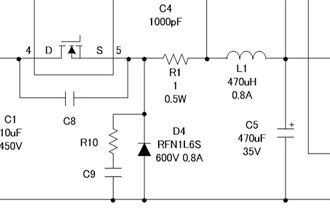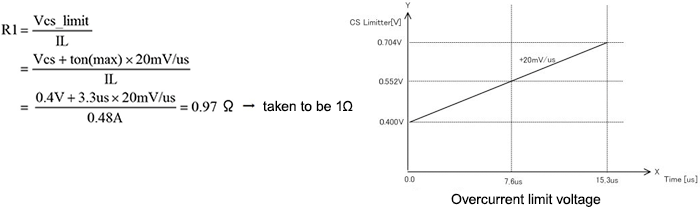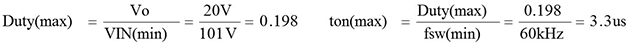Technical Information Site of Power Supply Design

• Basic Knowledge
• AC/DC
• Design Examples of Non-isolated Buck Converters
• Selecting Critical Components: Current Sense Resistor R1

2017.12.07 AC/DC

# Selecting Critical Components: Current Sense Resistor R1

Design Examples of Non-isolated Buck Converters

This is the third section on “Selecting Critical Components”. In the example circuit, a current sense resistor, mainly used to limit the switching current, is necessary. Selection of this current sense resistor is closely related to the “Inductor L1” of the previous section.

Current Sense Resistor R1

The circuit diagram on the right is excerpted from the example case. The current sense resistor R1 is placed in series in the line from the source of the internal MOSFET to the output. R1 limits the switching current, and provides protection against output overload, and is also used for slope compensation in current mode control.

Slope compensation is a well-known method to address harmonic oscillation in a current-mode step-down converter. Nearly all recent current-mode step-down converters are provided with a slope compensation circuit; compensation is achieved by using just a few external components such as a resistor. The power supply IC BM2P094F used in this circuit likewise employs a resistor R1.Harmonic oscillation is a phenomenon in which oscillation occurs with a period that is an integral multiple of the oscillation frequency. In a continuous-mode state, there is the risk of harmonic oscillation if the duty cycle is 50% or higher.

Calculation of the Current Sense Resistance R1

The current sense resistance R1 is calculated using the following equation. In this calculation, a number of the equations and values used in calculating the "inductance L1" of the previous section are necessary. In addition, information on the current-limiting characteristics specific to the power supply IC BM2P094F are also needed. We have already inserted the relevant values into the calculation equation and derived the solution.Here we explain the parameters used. R1 is the value of the overcurrent limit voltage Vcs_limit within the IC divided by the inductor peak current IL (Ip). Expanding Vcs_limit, the Vcs base is 0.4 V, to which is added a voltage rise that is proportional to a certain delay time from overcurrent detection. The graph in the upper-right is shown in the IC data sheet, and we see that CS_limit voltage increases by 20 mV for a delay time of 1 μs. From this, in the numerator of the calculation equation, 0.4 V is the base voltage, and 20 mV/μs is the rate of increase. As the delay time from overcurrent detection, the maximum switching-on time ton(max) is used.

ton(max) was calculated from the following equation when calculating the inductance in the previous section.The inductor peak current IL in the denominator was likewise determined in the preceding section. The maximum output current Iomax = 0.2 A × 1.2 = 0.24 A and the peak inductor current Ip = Iomax × 2 = 0.48 A are used.

In actual calculations, 0.4 V is added to 3.3 × 20 mV = 66 mV to obtain 0.466 V, which is divided by 0.48 A. According to Ohm's law, the resistance value is 0.97 Ω, which is rounded up to 1 Ω.

#### Key Points:

・The switching current limiting resistor R1 required in the example circuit is determined.

・In calculating R1, the numerical values used when calculating the inductance L1 are necessary.